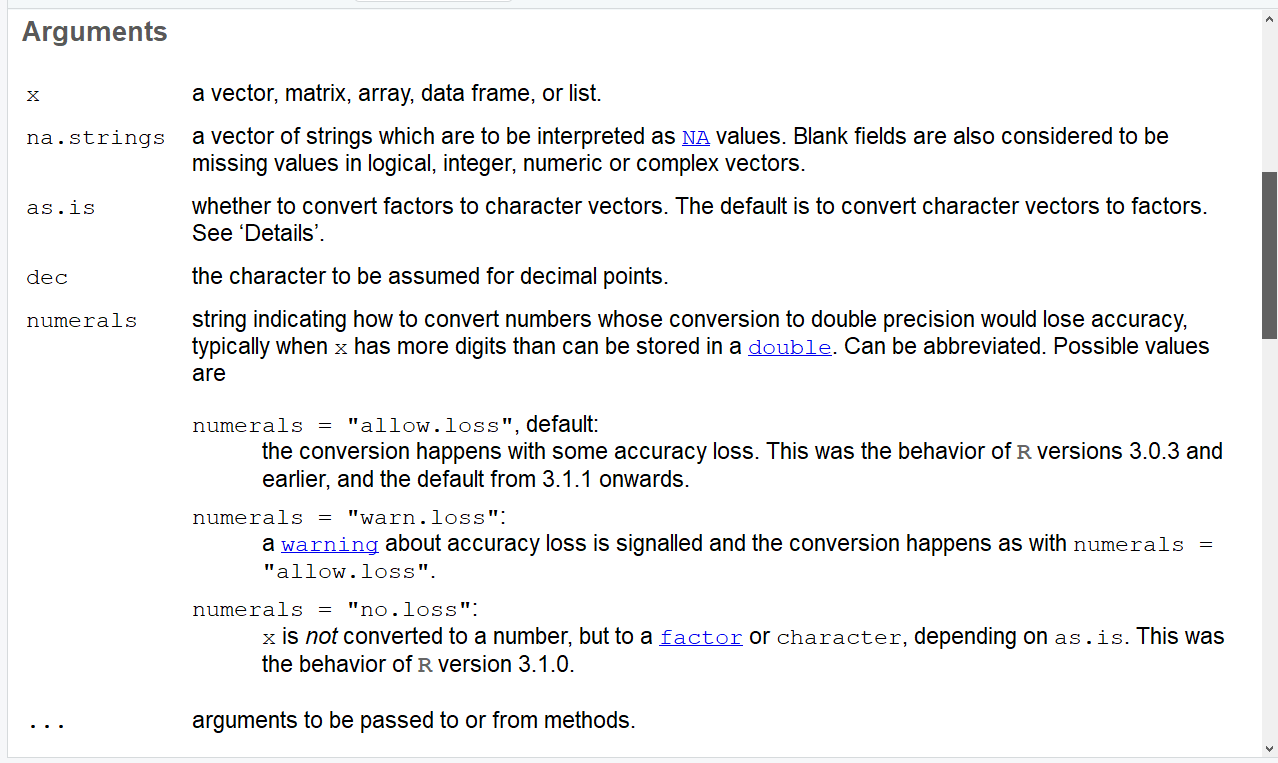# type.convert R Function (2 Examples) | Type Conversion in R Programming

In this tutorial you’ll learn how to apply the type.convert function in R.

Let’s have a look at the basic R syntax and the definition of the type.convert function first:

Basic R Syntax of type.convert():

`type.convert(x)`

Definition of type.convert():

The type.convert function computes the data type of a data object to an appropriate data type.

In the following, I’ll explain in two examples how to apply the type.convert command in R.

Let’s dive in!

## Example 1: Application of type.convert to Vector of Numbers

In this example we’ll apply the type.convert R function to a character vector that contains only numbers. Let’s create such a vector first:

`x1 <- c("7", "8", "4", "6", "1", "8", "1")  # Create example data`

AS you can see, our example vector contains only numbers. However, right now it has the class character:

```class(x1)                                   # Class of example data
# "character"```

We can now use the type.convert function to transform this character vector to a more appropriate class. Let’s see how this looks in practice:

`x1_convert <- type.convert(x1)              # Apply type.convert function`

And now let’s check the class of the converted vector:

```class(x1_convert)                           # Class of converted data
# "integer"```

As you can see, we just performed a type conversion from character to integer.

In other words: RStudio sees the integer class as most appropriate classification of our example vector. However, depending on the input vector, the data might be converted to other classes such as logical, numeric, complex, or factor.

Let’s see how the type conversion looks like, when we apply the type.convert function to a different vector…

## Example 2: Type Conversion of Character Vector Containing Numbers and Letters

Consider the following example data:

`x2 <- c(x1, "AAA")                          # Create second example data`

As you can see, our second example vector contains the same values as the vector used in Example 1, plus the character string “AAA”. Again, the original class is the character class:

```class(x2)                                   # Class of second example data
# "character"```

Now, let’s apply the type.convert function to this second example vector…

`x2_convert <- type.convert(x2)              # Apply type.convert function`

…and then let’s check the classification:

```class(x2_convert)                           # Class of converted data
# "factor"```

Even though we applied the type.convert function exactly the same way as in Example 1, we this time changed the class from character to factor.

This happened because R noticed the letters in the last element of the vector (i.e. “AAA”). Since such letters cannot be transformed to an integer or numeric, R selected the factor class as most appropriate.

## Video, Further Resources & Summary

I have also published a video tutorial on this topic, so if you are still struggling with the code, watch the following video on my YouTube channel:

Please accept YouTube cookies to play this video. By accepting you will be accessing content from YouTube, a service provided by an external third party.If you accept this notice, your choice will be saved and the page will refresh.

So far, I have shown you two different (and admittedly very simple) examples for the application of type.convert in the R programming language. However, there are many additional possibilities to modify the type.convert function. I recommend to have a look at the R help documentation of type.convert, in case you want to learn more about the function:Figure 1: R Help Documentation of type.convert().

Another thing you have to keep in mind is that the type.convert function is only one of many R functions that is able to change data classes. More precisely, you might also want to have a look at functions such as is.numeric, is.character, or is.factor.

Please accept YouTube cookies to play this video. By accepting you will be accessing content from YouTube, a service provided by an external third party.If you accept this notice, your choice will be saved and the page will refresh.

In addition, I can recommend to have a look at the other tutorials on my website. I have already written several tutorials on the conversion of different data classes:

In summary: I hope that you have a good basement for the transformation of different data types at this point. However, please let me know in the comments, in case you have any further questions.

Subscribe to the Statistics Globe Newsletter